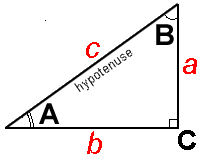Cleave Books
The Right-angled Triangles Calculator
 Show values to . . . 3 4 5 6 7 8 9 significant figures.
 edge a = units edge b = unitsedge c = units angle A = degrees angle B = degrees area = square units
Remember: Appropriate units need to be attached.
Very large and very small numbers appear in e-Format.
Unvalued zeros on all numbers have been suppressed.
The original inputs have NOT been adjusted in any way.
A note on Format and Accuracy is available.

 Additional Information The usual way of identifying a triangle is by first putting a capital letter on each vertex (or corner). Like, for example, A B C. Now, a reference to A can mean either that vertex or, the size of the angle at that vertex. Here it means the size.The edges are identified by using the small version of the opposite vertex-letter. So, a is opposite A;    b is opposite B;    c is opposite C. Again, the small letter could be identifying the edge or the length of the edge. Here it is the length. The name hypotenuse is given to the longest edge in a right-angled triangle. (It is the edge opposite to the right angle and is c in this case.) Where (for brevity) it says 'edge a', 'angle B' and so on, it should, more correctly, be something like 'length of edge a' or 'edge-length' or 'size of angle B' etc. If the perimeter is needed, add together the lengths ofedge a + edge b + edge c Note that giving the sizes of the two angles, A & B will not allow any other sizes to be found. At least one dimension, of edge-length or area, has to be supplied. Remember the drawing is NOT to scale. It is for illustration of the parts only. Sometimes two different solutions are possible. If one edge is known, and one angle; try putting in the edge length first as a and second as b; while putting in the angle as the SAME letter each time.

Go to Cleave Books main Index